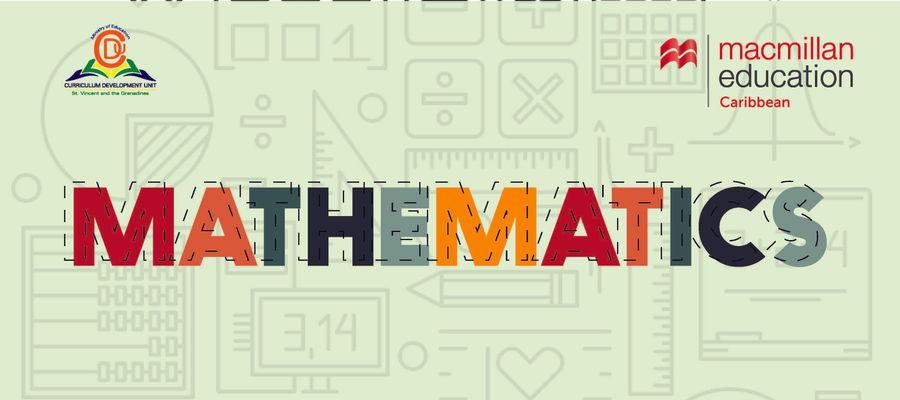# CPEA MOCK MATHEMATICS MCQ 4### Quiz Questions

• 1.
Express 6km 5m in metres
• A.
6005 m
• B.
6500 m
• C.
6050 m
• 2.
What is the perimeter of a square whose area is  144cm²?
• A.
12 cm
• B.
72 cm
• C.
48 cm
• 3.
An equilateral triangle has
• A.
all equal sides
• B.
two equal sides
• C.
no equal sides
• 4.
The mean of 6 numbers is 12. What is the total of the 6 numbers?
• A.
2
• B.
72
• C.
0.5
• 5.
Buses departs the bus terminal every 30 minutes. If the second bus left at 8:10 am. When did the FIRST bus leave the terminal?
• A.
7:40 am
• B.
8:40 am
• C.
7:30 am
• 6.
Troy watched a 96-minute football game between France and Switzerland. He watched the game for_____hrs____ minutes.
• A.
2hrs 6 mins
• B.
1hr 16 mins
• C.
1hr 36 mins
• 7.
Which of the following is an odd prime factor of 28?
• A.
2
• B.
4
• C.
7
• 8.
What is the value of 6 in 624?
• A.
6*10²
• B.
6*10¹
• C.
6*10⁰
• 9.
If 30% of a number is 12 .What is 50% of the same number
• A.
6
• B.
20
• C.
80
• 10.
The product of two numbers is 25. If one number is 0.5, what is the other number?
• A.
12.5
• B.
50
• C.
25.5

• 1.
Result 1